# Electrical Engineering test questions for exams and entrance - 10

## Electrical Engineering test questions for exams and entrance - 10

For Questions 1 and 2 refer to the data given below:

Let x = Ax + Bu, y = Cx be the state space equation of a system where x is the state vector, u is input and y is output.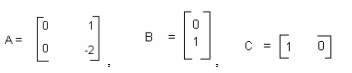(1) What will be the transfer function of this system?

(A) 1 / s(s+2)
(B) (s+1) / s(s+2)
(C) s / (s+1)
(D) s / (s+2)

(2) To make it a closed loop system a unity feedback is provided to the system described above, as shown in the figure.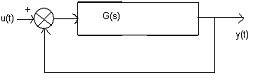What will be the steady state error in the output for a unit step input u (t)?

(A) 0
(B) 1
(C) ∞
(D) None of the above

For questions 3 and 4 refer to the data given below:

k G(s) = k(s+2) ² / s² (s-1)

(3) For stable operation the range of K will be

(A) -1(B) 0(C) 1(D) 2

(4) The imaginary axis crossover points is

(A) ± j1√2
(B) ± j√2
(C) ± j√5
(D) ± j2√2

(5) Which one of the following statement is true for the set of equations given below?

a1 + 2 a2 + a3 + 4 a4 = 2

3 a1 + 6 a2 + 3 a3 + 12 a4 = 6

(A) There are no solutions
(B) There are multiple non-trivial solutions.
(C) There is a unique non-trivial solution
(D) Only the trivial solution a1 = a2 = a3 = a4 = 0 exists.

ANSWER: There are multiple non-trivial solutions.

(6) In channel1 and channel2 of an oscilloscope, two in-phase, 50Hz sinusoidal waveforms of unit amplitude are fed. Assume voltage scale, time scale and other settings are exactly same

for both the channels. If the oscilloscope is operated in X-Y mode what will be observed?

(A) A straight line inclined at 45° with respect to x-axis
(B) A circle
(C) A parabola
(D) An ellipse

ANSWER: A straight line inclined at 45° with respect to x-axis

(7) Figure shows the periodic voltage waveform observed on an oscilloscope across the load. If a permanent magnet moving coil (PMMC) meter is connected across the same load then it will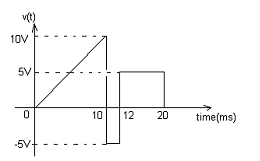(A) 8V
(B) 4V
(C) 1V
(D) 10V

(8) R1 = R2 =120 are the two fixed resistances of a single element strain gauge bridge. The bridge has a variable resistance, which gives full deflection at 120ohms at zero strain and at

120.6 ohms with a strain. What will be the value of strain in the beam if gauge factor of the strain gauge is 2?

(A) 0.0025
(B) 0.025
(C) 0.25
(D) 0.5

(9) Consider a 2 x 2 matrix whose trace is –2 and determinate is –35. The Eigen values are

(A) 2 and -4
(B) -4 and 6
(C) -1 and 2
(D) -7 and 5

(10) The phase crossover frequency for the transfer function

G(s)H(s) = 1/s(s+1)(s+0.5) is

(11) Which one of the following statement is true for the circuit turn-off time of an SCR?

(A) It is the time for which the SCR is reverse biased to reduce its current below the holding current.
(B) It is the time for which the SCR is reverse biased by the commutation circuit.
(C) It is the time taken by the SCR to turn off.
(D) It is the time required for the SCR current to become zero.

ANSWER: It is the time for which the SCR is reverse biased by the commutation circuit.

(12) RST instruction is an 8085 microprocessor system will cause an interrupt only if

(A) Interrupts are enabled by an EI instruction
(B) An interrupt service routine is not executed.
(D) None of the above

ANSWER: Interrupts are enabled by an EI instruction

(13) A transmission line has load impedance equal to characteristic impedance. The values of standing wave ratio and reflection coefficient are respectively

(A) 1 and 1
(B) 0 and 0
(C) 1 and 0
(D) 0 and 1

(14) Consider a lossless transmission line whose surge impedance loading (SIL) is 2280 MW. It is provided with a uniformly distributed series capacitive compensation of 30%. SIL of the

compensated transmission line will be

(A) 2000 MW
(B) 2725 MW
(C) 3000 MW
(D) 3725 MW

For Questions 15 and 16 refer to the data given below:

200V is applied to the armature of a separately excited DC motor that runs at a speed of 1500 rpm under no-load. The field voltage is maintained at its rated value. The rotational

loss and armature reaction are neglected. The figure shows that when the torque delivered by the motor is 5Nm, the speed of the dc motor is 1400rpm.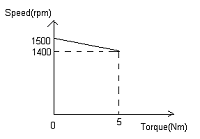(15) What is the armature resistance of the motor?

(A) 1.5Ω
(B) 4.5Ω
(C) 5.4Ω
(D) 3.4Ω

(16) When the torque to be delivered by the motor is 2.5Nm at 1400 rpm how much armature voltage should be applied?

(A) 184.5 V
(B) 120.6 V
(C) 150.1 V
(D) 193.3 V

(17) The race around condition in the circuit given below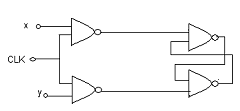(A) Occurs when CLK is 1 and input x and y are 0
(B) Occurs when CLK is 1 and input x and y are 1
(C) Occurs when CLK is 0
(D) Does not occur

(18) Match the following and select the correct option.
List I List II
Type of transmission line Type of distance relay preferred

i) Short line x) Ohm Relay
ii) Medium line y) Reactance Relay
iii) Long line z) MHO Relay

i) ii) iii)

(A) x z y
(B) x y z
(C) y x z
(D) z y x

(19) In the interval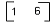the maximum value of f(x) = x³ - 9x² + 24x +5 is

(A) 41
(B) 28
(C) 36
(D) 16

(20) Consider the circuit shown below. What will be the voltage Vxy if we assume ideal elements in the circuit?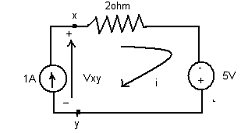(A) 0V
(B) 1V
(C) -3V
(D) 3V

(21) Consider an image that uses 12 x 512 picture elements. What will be the maximum entropy of the image when each of the picture elements can take any of the 8 distinguishable intensity

levels?

(A) 209165 bits
(B) 765439 bits
(C) 694 bits
(D) 144 bits

(22) The open loop system whose transfer function is given by

G(s) = (s–1)/ (s+2)(s+3) is

(A) Unstable and of the non-minimum phase type
(B) Stable and of the non-minimum phase type
(C) Unstable and of the minimum phase type
(D) Stable and of the minimum phase type

ANSWER: Stable and of the non-minimum phase type

(23) What will be the product of two matrices A and adj. A?

(A) Scalar matrix
(B) Unit matrix
(C) Zero matrix
(D) Matrix A itself

(24) In India picture and speech signals in commercial TV transmission are modulated respectively as

(A) VSB and FM
(B) FM and SSB
(C) SSB and VSB
(D) VSB and VSB

(25) Consider a 3-phase, star connected 15Kw, 4-pole, 230V, 50Hz induction motor. At rated conditions the locked rotor current is 50A. Losses and magnetizing current are negligible. When

the motor is connected to a 236V, 57Hz supply, the approximate locked rotor line current drawn is

(A) 50.2 A
(B) 34.8 A
(C) 45.0 A
(D) 25.9 A